# 15 triangles in a web of cubics

Consider a homogeneous cubic form in three variables,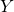, and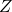, such as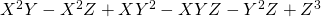Sometimes a cubic form can be factored. In this case, we are lucky: it factors as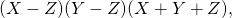but in general we will not be so lucky. It is pretty rare for a random cubic form to be factorable. From the perspective of projective algebraic geometry, a homogeneous cubic form cuts out an algebraic curve in the projective plane:

and a cubic that factors into three linear forms will cut out three lines: a “degenerate” plane cubic. Such a collection of three lines is called a “triangle”.

The space of homogeneous cubic forms is a 10-dimensional vector space with basis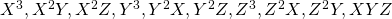. However, given a cubic form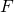, the scaled form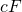corresponds to the same curve. Furthermore, if all the coefficients are zero, then the form doesn’t correspond to the curve at all. Thus the space of plane cubics is nine-dimensional projective space.

In this post we will prove the following enumerative result:

A general three-dimensional family of plane cubics contains exactly 15 triangles.

By a three-dimensional family (aka “web”), we mean some embedded copy of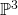in this space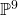of plane cubics. This geometric result corresponds to the following purely algebraic fact:

A general four-dimensional subspace of the ten-dimensional space of cubic forms contains exactly 15 forms which factor into three linear forms.

(I should probably say something about the term “general”. The statement “a general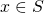satisfies property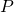” this means that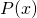says that the subset of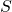for which P(x) holds is dense in.)

This material is drawn from 3264 And All That by Eisenbud and Harris

## The strategy

Let us consider the space of (ordered) triples of lines, or nonzero linear forms up to scaling. This is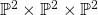. We can construct a morphism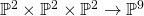which sends a triple of linear forms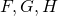to their product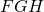. This map is (in general) 6 to 1, since there are 6 permutations of three (distinct) linear forms.

We will do intersection theory in: specifically, we will pull back the class of ainto, and count how many points it consists of (in other words, how many triples of linear forms correspond to cubic surfaces in a general web). Then we will divide this number by 6, to count the number of triangles in the family.

## Computation in the Chow ring

The morphism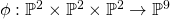induces a map of Chow rings: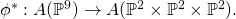Now,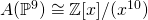and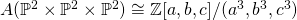. The class of anyin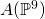is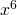. Furthermore,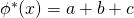. So,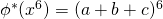. If we expand this out, removing every term that has any variable to a power of three or greater, we see that every term except the monomial term of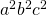vanishes, and its coefficient is the.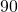is the the number of ordered triples of linear forms which correspond to cubic forms contained in a general web. Dividing by six, since six ordered triples correspond to one unordered triple (i.e. one distinct triangle), we obtain our answer of 15.

(I will add more details to this…)